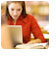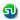### Logarithm, log Question, Answers. Math Prep. Ascent MBA TANCET, XAT, CAT, PGSEM Preparation, Correspondence course

 Home TANCET Classes GMAT Coaching Online GMAT Course CAT Classes TANCET Study Material
 TANCET '18 Classroom ProgramWeekend and weekday classes for TANCET MBA @ Chennai. At Nungambakkam and Velachery.Other Courses TANCET Correspondence Course CAT Classes & Courses GMAT Classes Chennai Online GMAT Course GRE Classes Chennai CBSE Math Online Tuition SAT Classes Other Links Ascent TANCET Toppers Testimonials CAT, TANCET Questions Careers @ Ascent Contact Us +91 44 4500 8484 +91 96000 48484 ascent@ascenteducation.com Postal Address Facebook / Twitter / Blog / Videos
You are here: Home  »  CAT, XAT, TANCET Prep Questions »  Miscellaneous Topics »   Logarithm

# Quantitative Reasoning : Logarithms

## Question

How many zeroes are there between the decimal point and the first significant digit in (1/9)200 given log103 = 0.4771?
1. 84
2. 85
3. 191
4. 190

Choice (4). 190 zeroes

## Note:

After taking log to the given number if the mantissa of the logarithm is negative, then the characteristic is equal to the number of zeroes immediately following the decimal point.

Therefore, log (1/9)200 = log (9-200) = -200 log (32) = -400 log (3) = -400(0.4771) = -190.84.

Hence, the number (1/9)200 will have 190 zeroes immediately after the decimal point.

## CAT, XAT, TANCET Practice Questions and Answers : Listed Topicwise

 Number Theory Permutation Combination Probability Inequalities Geometry Mensuration Trigonometry Coordinate Geometry Percentages Profit Loss Ratio Proportion Mixtures Alligation Speed Time Distance Pipes Cisterns Interest Races Average, Mean AP, GP, HP Set Theory Clocks Calendars Algebra Function English Grammar General Awareness Data sufficiency TANCET Papers XAT PapersAdd to del.icio.usStumble Itdigg this